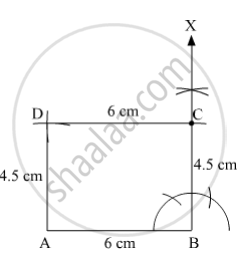SSC (English Medium) Class 8Maharashtra State Board
Share

# Draw a Rectangle Abcd Such that L(Ab) = 6.0 Cm and L (Bc) = 4.5 Cm. - SSC (English Medium) Class 8 - Mathematics

ConceptSome Special Parallelograms Concept of a Rectangle

#### Question

Draw a rectangle ABCD such that l(AB) = 6.0 cm and l (BC) = 4.5 cm.

#### Solution

Steps of Construction:

Step 1:
Draw AB = 6 cm.

Step 2: Draw ∠ABX = 90º.

Step 3: With B as centre and radius 4.5 cm, draw an arc cutting the ray BX at C.

Step 4: With C as centre and radius 6 cm, draw an arc.

Step 5: With A as centre and radius 4.5 cm, draw an arc cutting the previous arc at D.

Step 6: Join AD and CD.Here, ABCD is the required rectangle.

Is there an error in this question or solution?

#### APPEARS IN

Balbharati Solution for Balbharati Class 8 Mathematics (2019 to Current)
Chapter 8: Quadrilateral : Constructions and Types
Practice Set 8.2 | Q: 1 | Page no. 46
Solution Draw a Rectangle Abcd Such that L(Ab) = 6.0 Cm and L (Bc) = 4.5 Cm. Concept: Some Special Parallelograms - Concept of a Rectangle.
S